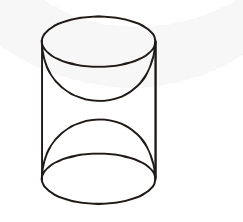# A wooden article was made by scooping out a hemisphere from each end of a solid cylinder, as shown in fig.Question:

A wooden article was made by scooping out a hemisphere from each end of a solid cylinder, as shown in fig. If the height of the cylinder is 10 cm, and its base is of radius 3·5 cm, find the total surface area of the article.Solution:

Radius of the cylinder (r) = 3.5 cm

Height of the cylinder (h) = 10 cm

$\therefore$ Curved surface area of cylinder $=2 \pi \mathrm{rh}$

$=2 \times \frac{22}{7} \times \frac{35}{10} \times 10 \mathrm{~cm}^{2}=220 \mathrm{~cm}^{2}$

Curved surface area of a hemisphere $=2 \pi \mathrm{r}^{2}$

$\therefore$ Curved surface area of both hemispheres

$=2 \times 2 \pi r^{2}=4 \pi r^{2}=4 \times \frac{\mathbf{2 2}}{\mathbf{7}} \times \frac{\mathbf{3 5}}{\mathbf{1 0}} \times \frac{\mathbf{3 5}}{\mathbf{1 0}} \mathrm{cm}^{2}=154 \mathrm{~cm}^{2}$

Total surface area of the remaining solid

$=(220+154) \mathrm{cm}^{2}=374 \mathrm{~cm}^{2}$